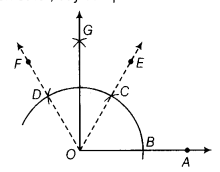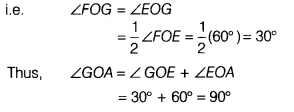# Construct an angle of 90° at the initial point of a given ray

Construct an angle of 90° at the initial point of a given ray.

(i) First, draw a ray OA.
(ii) Taking O as centre and some radius, draw an arc of circle which intersects OA, say at a point B.
(iii) Taking B as centre and with the same radius as before, draw an arc intersecting the previously drawn arc, say at a point C.
(iv) Taking C as centre and with the same radius as before, draw an arc intersecting the arc drawn in step (ii), say at D.
(v) Draw the dotted ray OE passing through C.
Then, ∠EOA = 60°
(vi) Draw the dotted ray OF passing through D.
Then, ∠FOE = 60°
(vii) Next, taking C and D as centres and with the radius more than 1/2 CD, draw arcs to intersect each other, say at a point G.(viii) Draw the ray OG. This ray OG is the bisector of the ∠FOE.